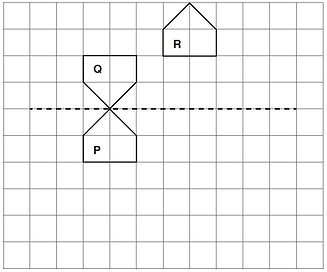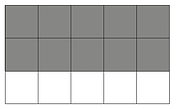top of page

### 11+ Mathematics ISEB 2016

1. Write down the answers to these questions.

(i) 48 + 35 = 83
(ii) 613 - 123 = 490
(iii) 28 / 4= 7
(iv) 2 3 = 8
(v) twenty-five percent of eighty = 20

(vi) 6.3 x 100 = 630
(vii) 398 + 297 = 695
(viii) 27 x 5 = 695

8 marks

2. (a) Write down all the prime numbers between 10 and 20

11, 13, 17, 19

2 marks

1 for 3 given or all correct but with 1 additional number

(b) Write down the first three multiples of 12

12, 24, 36

1 mark

(c) Write down all the factors of 16

1, 2, 4, 8, 16

2 marks

1 for 4 factors or all correct but 1 additional number

3. A box of 7 grapefruit costs £3.29

(i) What is the cost of one grapefruit? 47p

2 marks

Patrick buys 2 boxes of grapefruit and pays with a £20 note.

(ii) How much change should he receive? £13.42

2 marks

1 for £6.58 seen

4. These thermometers show the temperatures inside and outside a window at 10 a.m. one winter’s day.

(i) How many degrees warmer was it inside than outside the window? 24 °C

1 mark

At 10 p.m., the temperature outside had fallen by 2 °C.

(ii) What was the temperature outside the window at 10 p.m.? 25 °C

1 mark

5. Fill in the boxes to make the following statements true.

(i) 8 + 4 x 7 = 36

(ii) 5 x (4 - 1) = 15

(ii) 10 - (5 + 8) = -3

Total 3

6. Shape P is drawn on the centimetre-square grid below.

(i) Reflect shape P in the dashed line.

Label the new shape Q.

2 marks

1 for orientation, 1 for position

(ii) Translate shape P 3 units right and 4 units up.

Label the new shape R.

2 marks

1 for 3 units right, 1 for 4 units up(iii) Work out the area of shape P.

2 marks

1 for 3, 1 for cm2

7. (a) Write down the value of these Roman numerals.

(i) V = 5

(ii) M = 1000

(b) Which year is written in Roman numerals as MMXVII? 2017

1 mark for each

3 in total

8. (a) Work out the following.

(i) 3579 + 1824 = 5403

(ii) 3579 - 1824 = 1755

(iii) 264 x 27 = 7128

(iv) 1595 / 11 = 145

2 marks

2 marks

3 marks

(iii)1 for 1848, 1 for 5280, 1 for 7128

3 marks

(b) Which year is written in Roman numerals as MMXVII? 2017

1 mark

9. Calculate the mean of these numbers.

9 14 7 17 8 = 11

2 marks

1 for 55 seen

10. Choose from the list for fractions

(i) a fraction which is greater than 1  -  15/11

(ii) a fraction equivalent to 80%  -   4/5

(iii) a fraction equivalent to 0.75  -  3/4

(iv) a fraction which is not in its simplest form   -   8/12

1 marks for each

4 in total

11. A sunflower is 150 cm tall.

How tall will it be if its height increases by 10%? 165 cm

1 mark

12. Refer to the 5 number cards:

(i) Using all 5 cards

(a) what is the largest possible even number?   97536

(b) what is the number which is closest to 80 000?   79653

2 marks

1 for each

(ii) Use exactly 2 of the cards to make the smallest possible prime number.

37

1 mark

(iii) Arrange any 4 of the cards to show a sum below which will give the smallest possible answer.

36 + 57 or 37 + 56

1 mark

1 in either order

13. Put these distances in order from smallest to largest.

Smallest - 2.087 km, 2.708 km, 2778 m, 27.8 km - Largest

3 marks

1 for converting units, 2 allow each answer any unit, subtract one for each error or omission

14. (i) Draw accurately triangle ABC where AB 5 5.5 cm, angle A 5 45° and angle B 5 90°

(i) Reflect shape P in the dashed line.

Label the new shape Q.(ii) Measure and write down the length of side BC. 5.5 cm

(iii)  Circle any appropriate words from the box above to describe triangle ABC.

Isosceles, Right-angled

Reasons: angle B is 90° and AB 5 BC or angle C 5 45° 5 angle A

3 marks

1 for angle A 5 45 ± 2°

1 for AB 5 5.5 ± 0.1 cm and angle B 5 90 ± 2°

1 for completing triangle

1 mark

Allow 5.4 cm - 5.6 cm

2 marks

1 for both circled. 1 for reasoning.

15. (i) Two identical rectangles are divided into 15 equal squares.

(a) Shade 3/5 of this rectangle:(a) Shade 2/3 of this rectangle:

(c) Which is larger: 2/3 or 2/5. Give your reasons.

2/3 because ....

e.g 2/3 = 10/15 and 3/5 = 9/15

3

5

2

3

4

5

13

15

1

3

1

1 mark

1 for any 9 squares shaded

1 mark

1 for any 10 squares shaded

(ii) Arrange these fractions in order from smallest to largest.

1 mark

1 for 2/3

1 for reason

2 marks

2 subtract 1 for each error or omission

16. Work out?

(i) 2/3 - 1/6 =

1/2

1  1/5

2 marks for each

4 in total

17. In a box of 24 pens, one eighth are green, 25% are red and the rest are blue.

What fraction of the pens is blue? 5/8

3 marks

1 for green, 1 for 6 red, 1 for answer

18. (a) Jake hangs a peg basket on a washing line.

Find the size of one of the shaded angles in the diagram above, if both are the same size. 110°

2 marks

1 for 220°

(b) The diagram below shows two straight lines. Find the sizes of the angles marked a, b and c.

a = 5 30°

b = 5 150°

c = 5 150°

3 marks

19.  Sarah measured the temperature of a beaker of liquid every 10 minutes during a science experiment.

(i) What was the lowest temperature of the beaker? 10 °C

(ii) At what time was the temperature of the beaker 15 °C? 10:30 a.m.

Sarah measured the temperature of the beaker again 4 hours and 30 minutes after the last reading on the graph.

(iii) At what time did she take this measurement? 3:20 p.m.

3 marks

20. Farmer Jack and Farmer Giles each have a rectangular field. Farmer Jack’s field has an area of 24 m2. Its length is 8 m.

(i) Work out the width of Farmer Jack’s field. 3 m

(ii) Work out the perimeter of Farmer Jack’s field. 22 m

Farmer Giles’ field has a perimeter of 20 m. The width of Farmer Giles’ field is 4 m.

(iii) Work out the length of Farmer Giles’ field. 6 m

(iv) Work out the area of Farmer Giles’ field. 24 m2

Farmer Josephine also has a rectangular field. The width of Farmer Josephine’s field is x m and the length is y m. Farmer Josephine builds a fence along the perimeter of her field.

(v) If the total length of this fence is 30 m, write down two possible values of x and y.

e.g. x 5 5 m and y 5 10 m

or x 5 6 m and y 5 9 m

2 marks for each

(v)1 for each pair values which sum to 15

21. Sanjay is making purple paint.

(i) If he uses 6 litres of red paint, how much blue paint should he use? 9 litres

(ii) How much blue paint is needed to make 35 litres of purple paint? 21 litres

1 mark for each

2 marks in total

22. (a) Annie and Bradley each think of a number. The difference between their numbers is 6 The sum of their numbers is 20

What are the two numbers? 13 and 7

1 mark

(b) Alice thinks of a number. Alice calls her number a. Alice adds 7 to her number, and then doubles her answer. .

Write an expression, using a, to show what Alice does. 2(a + 7)

2 marks

1 for a 1 7

1 for multiplying by 2 accept correct equivalent form

e.g. (a + 7) x 2 or 2a + 14

(c) Jack thinks of a number. Jack calls his number n. Jack multiplies his number by 3, and then subtracts 5. He gets the answer 16

Use this information to write down an equation, and then solve it to find n. n = 7

1 mark

1 for 3n - 5 = 16

1 for n 5 7 (allow this mark even if equation has not been formed)

bottom of page